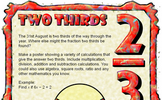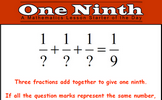# Fractions

### Term 1 starting in week 8 :: Estimated time: 4 weeks

• Equivalent fractions (review)
• Simplify fractions
• Improper fractions to mixed numbers (review)
• Mixed numbers to improper fractions (review)
• Fractions on a number line
• Compare and order (denominator)
• Compare and order (numerator)
• Add and subtract fractions (1)
• Add and subtract fractions (2)
• Subtract mixed numbers (review)
• Subtract fractions
• Multiply fractions by integers
• Multiply fractions by fractions
• Divide fractions by integers (1)
• Divide fractions by integers (2)
• Four rules with fractions
• Fraction of an amount
• Fraction of an amount — find the whole

This page should remember your ticks from one visit to the next for a period of time. It does this by using Local Storage so the information is saved only on the computer you are working on right now.

## Lesson Starters

Here are some suggestions for whole-class, projectable resources which can be used at the beginnings of each lesson in this block.

### 1st Lesson#### Coins On The Table

A puzzle about the number of coins on a table given information about fractions of them.

### 2nd Lesson#### Fractangle

Can you see what fractions of the shapes have been shaded? It is not as difficult as it first appears.

### 3rd Lesson#### Giraffe

The height of this giraffe is three and a half metres plus half of its height. How tall is the giraffe?

### 4th Lesson#### Halloween

Five problems with a Halloween theme.

### 5th Lesson#### Inbetweens

Find fractions between two given values.

### 6th Lesson#### Two Thirds

Make a poster showing a variety of calculations that give the answer two thirds.

### 7th Lesson#### One Ninth

Investigate three fractions which add together to give one ninth.

### 8th Lesson#### Santa's Sleigh

Work out the number of clowns and horses given the number of heads and feet.

### 9th Lesson#### Wrapping Paper

Find the order of rotational symmetry of the repeating pattern.

### 10th Lesson#### Boxing Day

Work out the contents and the cost of the Christmas boxes from the given clues.

### 11th Lesson#### Christmas Eve

Is there a pattern in the number of palindromic numbers to be found less than powers of 10?

### 12th Lesson#### Inbetween Table

Write down as many multiples of 3.5 as possible in 3.5 minutes.

### 13th Lesson#### Ropey Snowballs

Arrange the numbers on the snowballs so that no two consecutive numbers are directly connected by rope.

Some of the Starters above are to reinforce concepts learnt, others are to introduce new ideas while others are on unrelated topics designed for retrieval practice or and opportunity to develop problem-solving skills.

White Rose ResourcesEnd of block assessments provide a quick progress check at the end of each block of learning to make sure students have understood the content covered. This Scheme of Learning was produced by White Rose Maths and is used here with permission granted on 30th June 2021.For All: